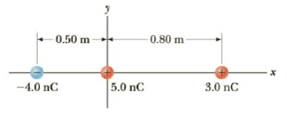Chapter 15, Problem 57AP

Chapter
Section
Textbook Problem

Three point charges are aligned along the x-axis as shown in Figure P15.57. Find the electric field at the position x = +2.0 m, y = 0.Figure P15.57

To determine
The electric field at 2.0 m.

Explanation

The electric field directions are given by,

• E1 , E2 and E3 are electric field due to the charges q1 , q2 and q3 .

The total electric field is,

E=E2+E3E1

Formula to calculate the total electric field at the point is,

E=keq2r22+keq3r32keq1r12

• ke is the Coulomb constant.
• r1 , r2 and r3 are the distances between q1 , q2 , q3 and the point.

Substitute 8.99×109 Nm2/C2 for ke , 2.5 m for r1 , 2.0 m for r2 and 1.2 m for r3 , 4.0 nC for q1 , 5.0 nC for q2 and 3.0 nC for q3

Still sussing out bartleby?

Check out a sample textbook solution.

See a sample solution

The Solution to Your Study Problems

Bartleby provides explanations to thousands of textbook problems written by our experts, many with advanced degrees!

Get Started